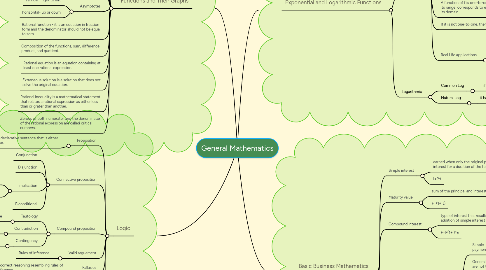# General Mathematics

Get Started. It's FreeGeneral Mathematics## 1. Functions and Their Graphs

### 1.9. Asymptotes

1.9.1. vertical - right to left

1.9.2. horizontal- up or down

## 2. Exponential and Logarithmic Functions

### 2.1. Exponential

2.1.1. If f is increasing or decreasing throughout domain, then f is one-to-one

2.1.2. if each horizontal line intersects the graph in at most one point, then the function if one-to-one.

2.1.3. Laws of exponent

2.1.3.1. (a^x)(a^y)=a^x+y

2.1.3.2. (a^x)^Y=a^xy

2.1.3.3. (ab)^x=a^x b^x

2.1.3.4. (a/b)^x= (a^x)/(b^x)

2.1.3.5. (a^x)/(a^y)=a^x-y

2.1.4. A function of f is one-to-one if every number in its range  corresponds to exactly one number i its domain.

2.1.5. If it is not one-to-one, then their is no inverse.

2.1.6. Real Life applications

2.1.6.1. Half-life decay model

2.1.6.2. Compound interest

2.1.6.3. Doubling time growth

2.1.6.4. Population growth

### 2.2. Logarithmic

2.2.1. Common Log

2.2.1.1. if there is no base, the base will automatically 10

2.2.2. Natural Log

2.2.2.1. it has a base of e written as lnx

### 3.1. Simple interest

3.1.1. earned when only the original principal earns interest for a duration of the term.

3.1.2. I=Prt

### 3.2. Maturity value

3.2.1. sum of the principal and interest rate.

3.2.2. F=(1+rt)

### 3.3. Compound interest

3.3.1. type of interest that results from the periodic addition of simple interest to principal.

3.3.2. F=P(1+i)^n

### 3.4. Annuity -  sequence of equal payment

3.4.1. Simple - interest period is the same as the payment period.

3.4.2. General - interest period and payment period are not the same.

3.4.3. - the payments are made are made at the end of each interest period.

3.4.4. Deffered - the first payment is not made at the end of the first interest period but later.

### 3.5. Stocks

3.5.1. shares of ownership in a company

### 3.6. Bonds

3.6.1. is a loan.

3.6.2. for corporations to raised the necessary funds.

### 3.7. Amortization

3.7.1. dept-repayment scheme wherein the original amount borrowed is repaid by making equal payments periodically.

### 3.8. Outstanding principal

3.8.1. dept that are still unpaid.

## 4. Logic

### 4.1. Proposition

4.1.1. Complete declarative sentence that is either true or false.

### 4.2. Connective proposition

4.2.1. Conjunction

4.2.2. Disjunction

4.2.3. Implication

4.2.3.1. Hypothesis and Conclusion

4.2.3.2. logically equivalent to its contrapositive

4.2.4. Biconditional

### 4.3. Compound proposition

4.3.1. Tautology

4.3.1.1. always true

4.3.2.1. Always false

4.3.3. Contingency

### 4.4. Valid arguement

4.4.1. Rules of inference

### 4.5. Fallasies

4.5.1. incorrect reasoning resembling rules of inference.

### 4.6. Method of proofs

4.6.1. Direct

4.6.2. Indirect

4.6.3. Existence

4.6.4. Nonexistence